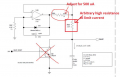Help me understand this current source

robotDR

Joined Mar 17, 2020
36
Hi All,

Thank you for checking this out. I was asked to add a current source to a circuit, and this schematic snippet was presented to me.

My questions are:
1) if this was originall a ramp circuit, which is the appropriate output for a current source? After R98?
2) Should R99 go to ground?
3) it says 375.94 uA = 2.5V/6.65kohm. That arrow below the 4 point junction. Why isn't R99 taken into consideration?
Thank you for looking. I appreciate your time.Irving

Joined Jan 30, 2016
2,416
The opamp maintains a constant 2.5v across the 6.65k resistor. This current flows through R99 to C124 which charges linearly because the current is constant. R99 has no impact on the current as long as the op amp output voltage plus 2.5V plus the voltage drop over R99 is sufficient to allow C124 to charge to the maxiumum desired voltage, which appears to be 5v. So the supply voltage to U48 must be >> 5 + 2.5 + R99*376uA = 8.75V The LM7301 on a 9v or higher rail meets this requirement.

•robotDR

robotDR

Joined Mar 17, 2020
36
The opamp maintains a constant 2.5v across the 6.65k resistor. This current flows through R99 to C124 which charges linearly because the current is constant. R99 has no impact on the current as long as the op amp output voltage plus 2.5V plus the voltage drop over R99 is sufficient to allow C124 to charge to the maxiumum desired voltage, which appears to be 5v. So the supply voltage to U48 must be >> 5 + 2.5 + R99*376uA = 8.75V The LM7301 on a 9v or higher rail meets this requirement.
Thank you Irving, that was wonderfully clear.

I am adapting this circuit to serve as a current bias for a thermistor. Do I still need C124? Do I output to the thermister between R98 and R99 or after R99?

Irving

Joined Jan 30, 2016
2,416
If you're using a standard 10k thermistor then use that instead of R99 and C124. That will give the following voltages:
-20degC: 78.9k * 376uA = 29.7V
0degC: 29.5k * 376uA = 11.1V
25degC: 10k * 376uA = 3.76V
40degC: 5.8k *376uA = 2.18v
100degC: 816Ω * 376uA = 0.3v

Clearly 0 - 100C is possible with a 12v supply. If you want to go much below zero you'll need to reduce the current and/or increase the voltage, or choose a different thermistor.

•robotDR